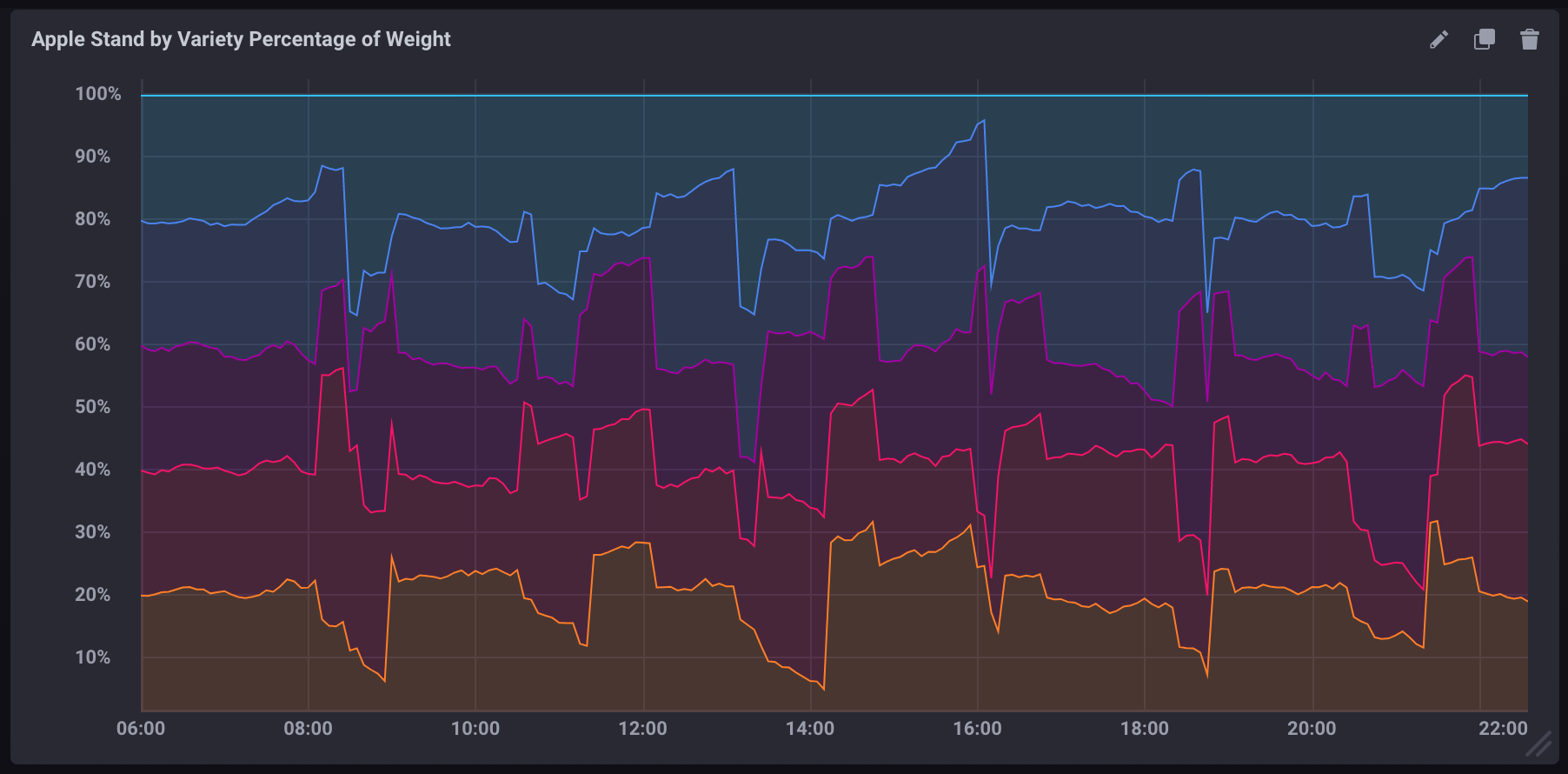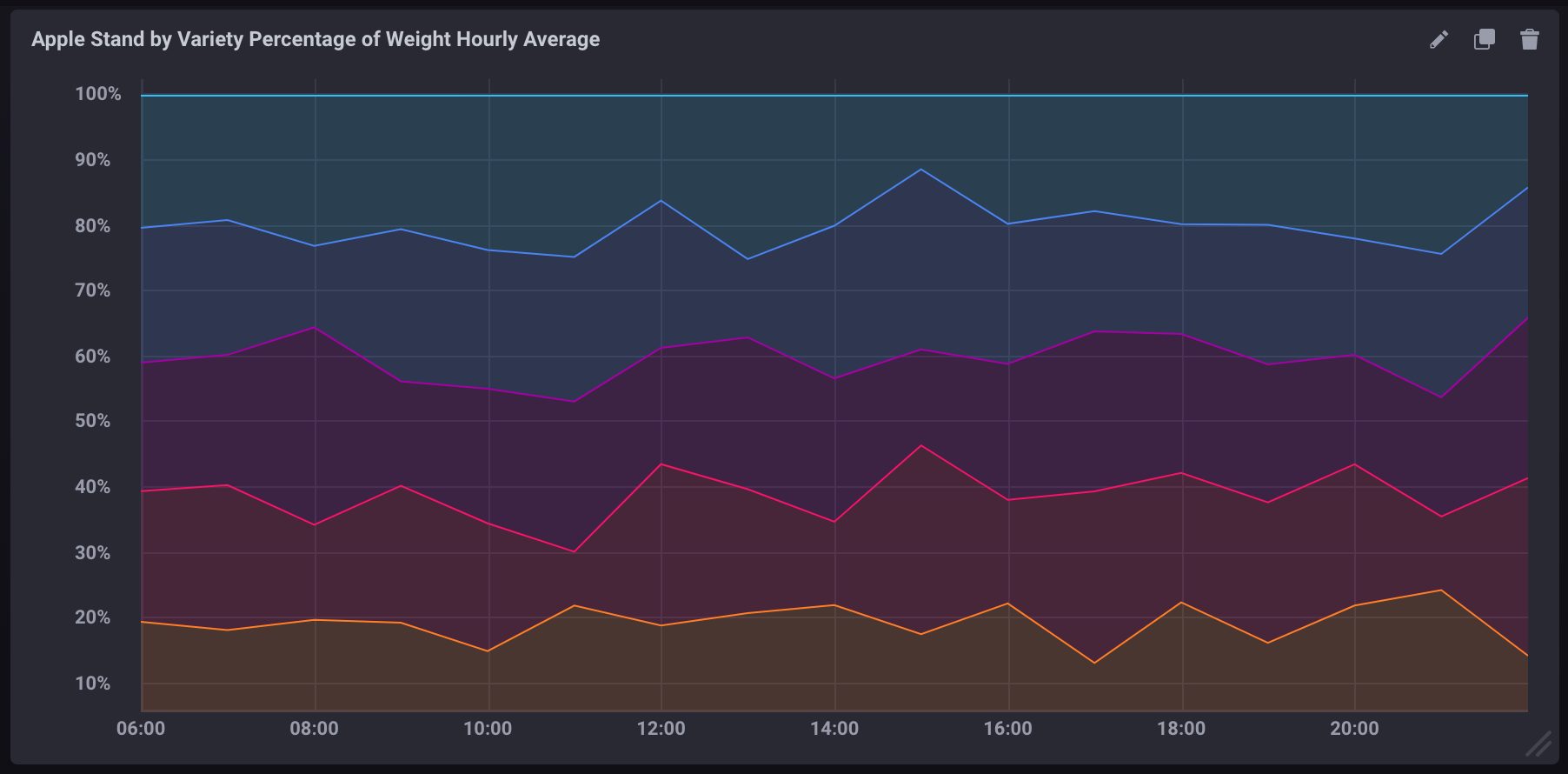# Calculating percentages in a query

This page documents an earlier version of InfluxDB. InfluxDB v2.3 is the latest stable version.

InfluxQL lets you perform simple math equations which makes calculating percentages using two fields in a measurement pretty simple. However there are some caveats of which you need to be aware.

## Basic calculations within a query

`SELECT` statements support the use of basic math operators such as `+`,`-`,`/`, `*`, `()`, etc.

``````-- Add two field keys
SELECT field_key1 + field_key2 AS "field_key_sum" FROM "measurement_name" WHERE time < now() - 15m

-- Subtract one field from another
SELECT field_key1 - field_key2 AS "field_key_difference" FROM "measurement_name" WHERE time < now() - 15m

-- Grouping and chaining mathematical calculations
SELECT (field_key1 + field_key2) - (field_key3 + field_key4) AS "some_calculation" FROM "measurement_name" WHERE time < now() - 15m
``````

## Calculating a percentage in a query

Using basic math functions, you can calculate a percentage by dividing one field value by another and multiplying the result by 100:

``````SELECT (field_key1 / field_key2) * 100 AS "calculated_percentage" FROM "measurement_name" WHERE time < now() - 15m
``````

## Calculating a percentage using aggregate functions

If using aggregate functions in your percentage calculation, all data must be referenced using aggregate functions. You can’t mix aggregate and non-aggregate data.

All Aggregate functions need a `GROUP BY time()` clause defining the time intervals in which data points are grouped and aggregated.

``````SELECT (sum(field_key1) / sum(field_key2)) * 100 AS "calculated_percentage" FROM "measurement_name" WHERE time < now() - 15m GROUP BY time(1m)
``````

## Examples

#### Sample data

The following example uses simulated Apple Stand data that tracks the weight of baskets containing different varieties of apples throughout a day of business.

2. Import the sample data:
``````influx -import -path=path/to/apple_stand.txt -precision=s -database=apple_stand
``````

### Calculating percentage of total weight per apple variety

The following query calculates the percentage of the total weight each variety accounts for at each given point in time.

``````SELECT
("braeburn"/total_weight)*100,
("granny_smith"/total_weight)*100,
("golden_delicious"/total_weight)*100,
("fuji"/total_weight)*100,
("gala"/total_weight)*100
FROM "apple_stand"."autogen"."variety"
``````
\*

If visualized as a stacked graph in Chronograf, it would look like:### Calculating aggregate percentage per variety

The following query calculates the average percentage of the total weight each variety accounts for per hour.

``````SELECT
(mean("braeburn")/mean(total_weight))*100,
(mean("granny_smith")/mean(total_weight))*100,
(mean("golden_delicious")/mean(total_weight))*100,
(mean("fuji")/mean(total_weight))*100,
(mean("gala")/mean(total_weight))*100
FROM "apple_stand"."autogen"."variety"
WHERE time >= '2018-06-18T12:00:00Z' AND time <= '2018-06-19T04:35:00Z'
GROUP BY time(1h)
``````

• It uses aggregate functions (`mean()`) for pulling all data.
• It includes a `GROUP BY time()` clause which aggregates data into 1 hour blocks.
• It includes an explicitly limited time window. Without it, aggregate functions are very resource-intensive.

If visualized as a stacked graph in Chronograf, it would look like: